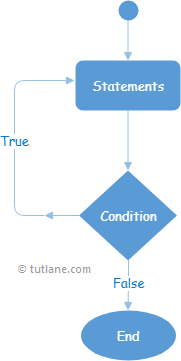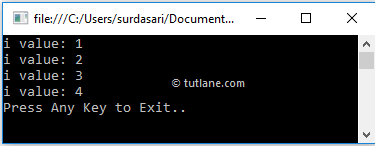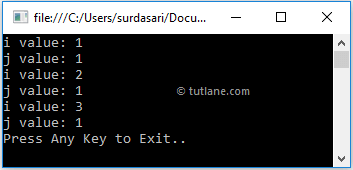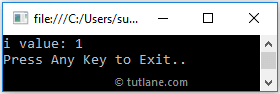# Visual Basic (VB) Do While Loop

In c#, the Do-While loop is useful to execute the block of statements until the defined condition returns true.

In Visual Basic, the do-while loop is same as the while loop, but the only difference is while loop will execute the statements only when the defined condition returns true, the do-while loop will execute the statements at least once because first it will execute the block of statements and then it will checks the condition.

## Visual Basic Do-While Loop Syntax

Generally, in Visual Basic Do and While keywords are useful to create a do...while loop. Following is the syntax of defining a do-while loop in Visual Basic programming language to execute the block of statements till the defined condition evaluates as false.

Do
// Statements to Execute
Loop While boolean_expression

If you observe the above syntax, the Do-While loop starts with the Do keyword followed by the block of statements and While with a parameter called boolean_expression.

Here, the statements of the Do-While loop will execute first; after that, the boolean_expression will be evaluated. If boolean_expression returns true, the statements inside the Do-While loop will execute again.

If boolean_expression is false, the Do-While loop will stop the execution of statements, and the program execution will come out of the loop.

## Visual Basic Do While Loop Flow Chart

Following is the pictorial representation of the Do-While loop process flow in the Visual Basic programming language.Now, we will see how to use the Do-While loop in the Visual Basic programming language with examples.

## Visual Basic Do While Loop Example

Following is the example of using a Do-While loop in Visual Basic programming language to execute the block of statements based on our requirements.

Module Module1
Sub Main()
Dim i As Integer = 1
Do
Console.WriteLine("i value: {0}", i)
i += 1
Loop While i <= 4
Console.WriteLine("Press Enter Key to Exit..")
End Sub
End Module

If you observe the above example, first, we are executing the statements within the Do-While loop and increasing the variable i (i++) value to 1 using the increment operator.

After that, the condition (i <= 4) will be evaluated, and again it will execute the block of statements if the defined condition returns true otherwise, it will terminate the loop.

When we execute the above Visual Basic program, we will get the result as shown below.If you observe the above result, the Do-While loop has been executed until it matches the defined condition (i <= 4). The program execution came out of the loop whenever the defined condition returned false.

## Visual Basic Nested Do-While Loop

In Visual Basic, we can use one Do-While loop within another Do-While loop to implement the application based on our requirements.

Following is the example of implementing a nested Do-While loop in Visual Basic programming language.

Module Module1
Sub Main()
Dim i As Integer = 1
Do
Console.WriteLine("i value: {0}", i)
i += 1
Dim j As Integer = 1
Do
Console.WriteLine("j value: {0}", j)
j += 1
Loop While j < 2
Loop While i < 4
Console.WriteLine("Press Enter Key to Exit..")
End Sub
End Module

If you observe the above example, we used one Do-While loop within another Do-While loop to achieve our application's nested do-while loop functionality based on our requirements.

When we execute the above Visual Basic program, we will get the result as shown below.If you observe the above result, both do-while loops were executed and returned based on our requirements.

## Visual Basic Do-While Loop with Exit Statement

In Visual Basic, we can exit or terminate the execution of the Do-While loop immediately by using `Exit` keyword.

Following is the example of using `Exit` keyword in the Do-While loop to terminate the loop execution in Visual Basic programming language.

Module Module1
Sub Main()
Dim i As Integer = 1
Do
Console.WriteLine("i value: {0}", i)
i += 1
If i = 2 Then Exit Do
Loop While i < 4
Console.WriteLine("Press Enter Key to Exit..")
End Sub
End Module

If you observe the above example, whenever the variable (i) value become 2, we are terminating the loop using `Exit` statement.

When we execute the above Visual Basic program, we will get the result as shown below.This is how we can use the `Exit` statement with the Do-While loop to terminate the loop execution based on our requirements.